# ELMo Contextual Word Representations Trained on1B Word Benchmark

Represent words as contextual word-embedding vectors

Released in 2018 by the research team of the Allen Institute for Artificial Intelligence (AI2), this representation was trained using a deep bidirectional language model. It produces three vectors per token, two of which are contextual, meaning that they depend on the entire sentence in which they are used. These word vectors are aimed at being linearly combined. They are based on the characters and case-sensitive, so there is no token dictionary.

Number of layers: 127 | Parameter count: 93,600,864 | Trained size: 375 MB |

## Examples

### Resource retrieval

Get the pre-trained net:

 In:=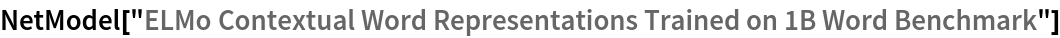Out=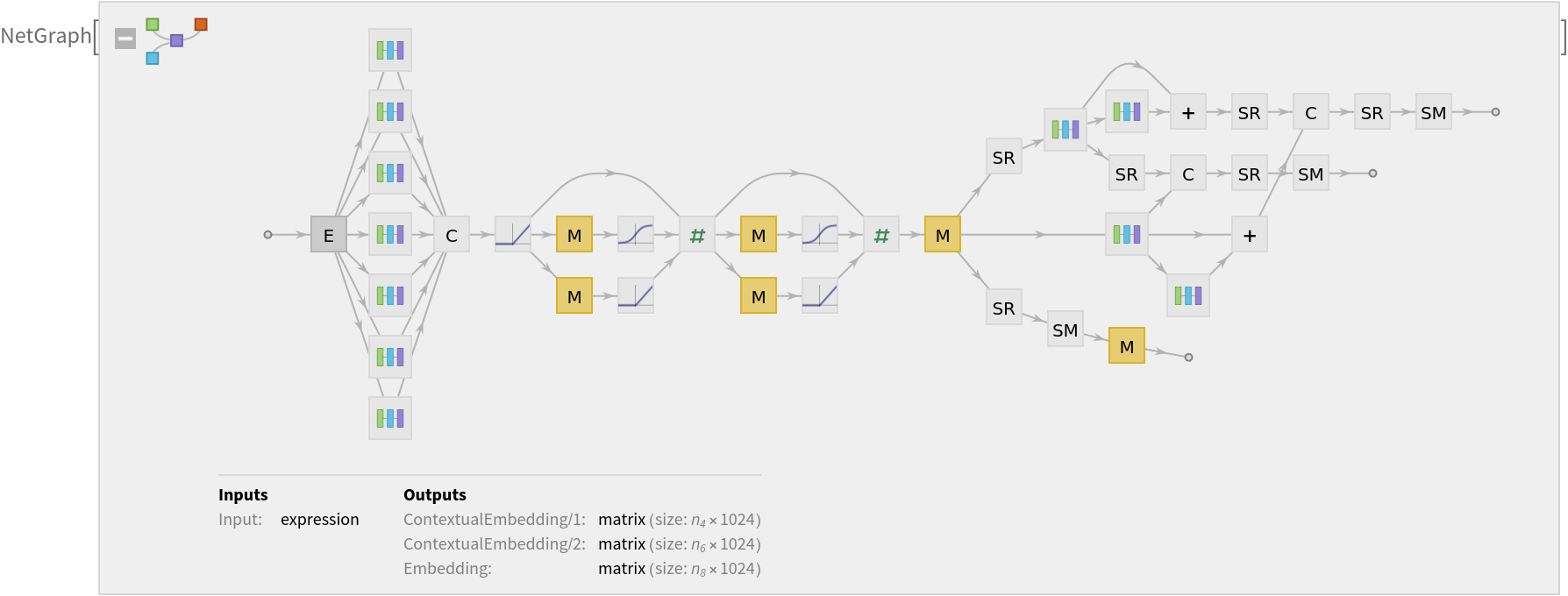### Basic usage

For each token, the net produces three length-1024 feature vectors: one that is context-independent (port "Embedding") and two that are contextual (ports "ContextualEmbedding/1" and "ContextualEmbedding/2").

Input strings are tokenized, meaning they are split into tokens that are words and punctuation marks:

 In:=Out=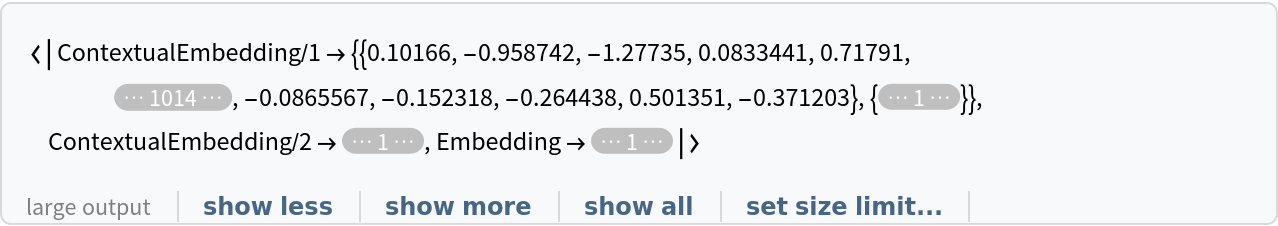Pre-tokenized inputs can be given using TextElement:

 In:=Out=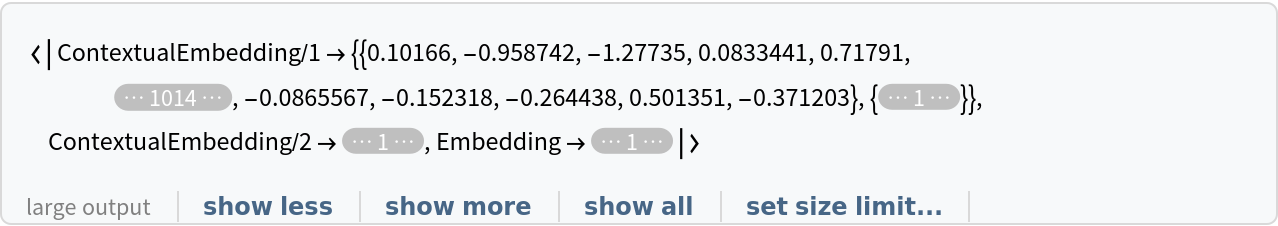The representation of the same word in two different sentences is different. Extract the embeddings for a different sentence:

 In:=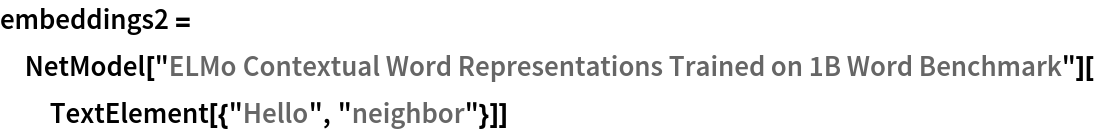Out=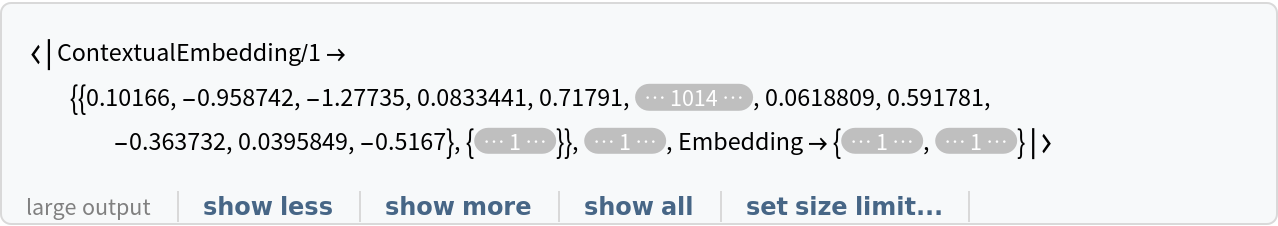The context-independent embedding for the same word is the same, whatever the surrounding text is. For instance, for the word "Hello":

 In:=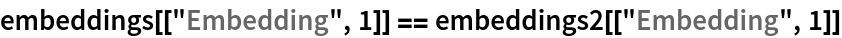Out=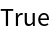The context-dependent embeddings are different for the same word in two different sentences:

 In:=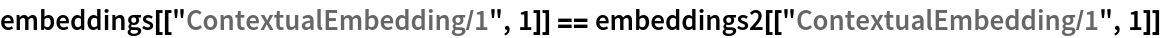Out=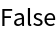In:=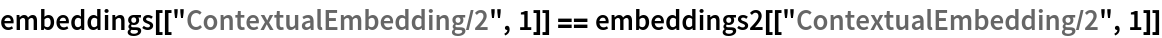Out=The recommended usage is to take a (possibly weighted) average of the embeddings:

 In:=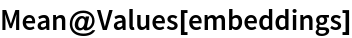Out=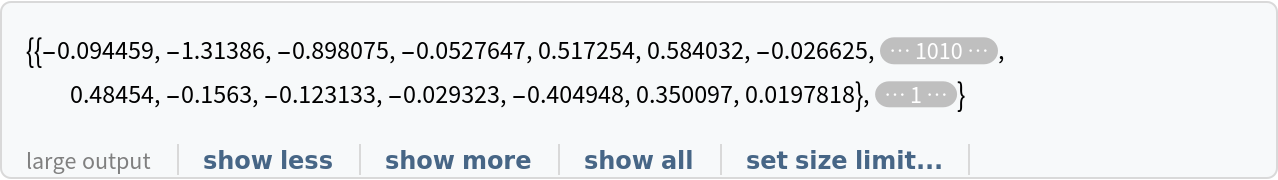### Word analogies without context

Extract the non-contextual part of the net:

 In:=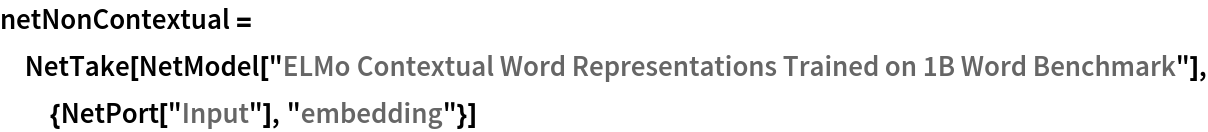Out=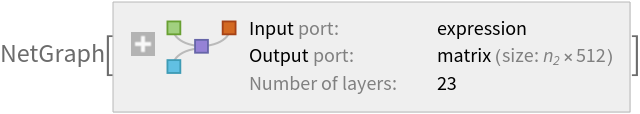Precompute the context-independent embeddings for a list of common words (if available, set TargetDevice -> "GPU" for faster evaluation time):

 In:=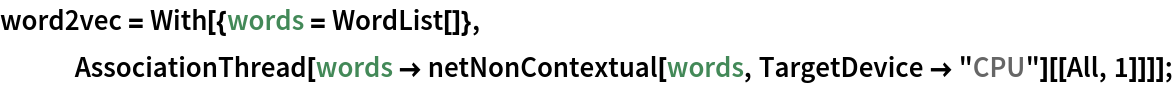Find the five nearest words to "king":

 In:=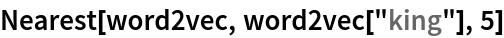Out=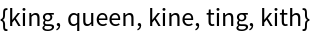Man is to king as woman is to:

 In:=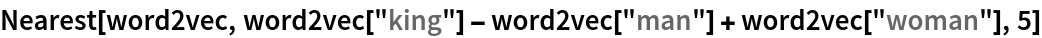Out=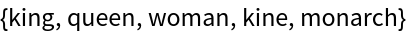Visualize the similarity between the words using the net as a feature extractor:

 In:=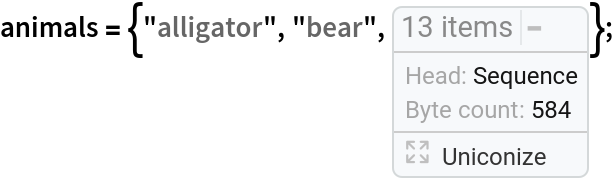In:=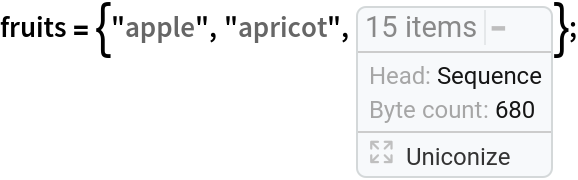In:=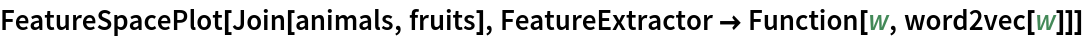Out=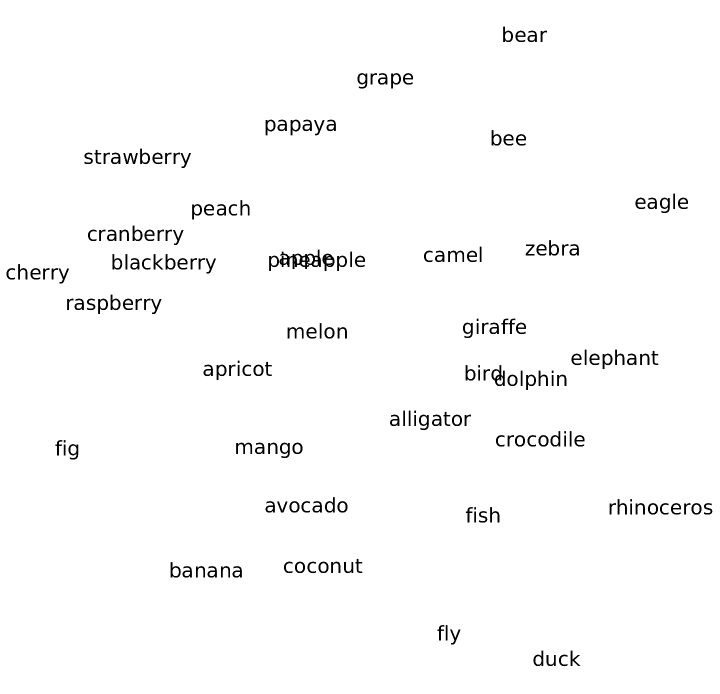### Word analogies in context

Define a function that shows the word in context along with the average of its embeddings:

 In:=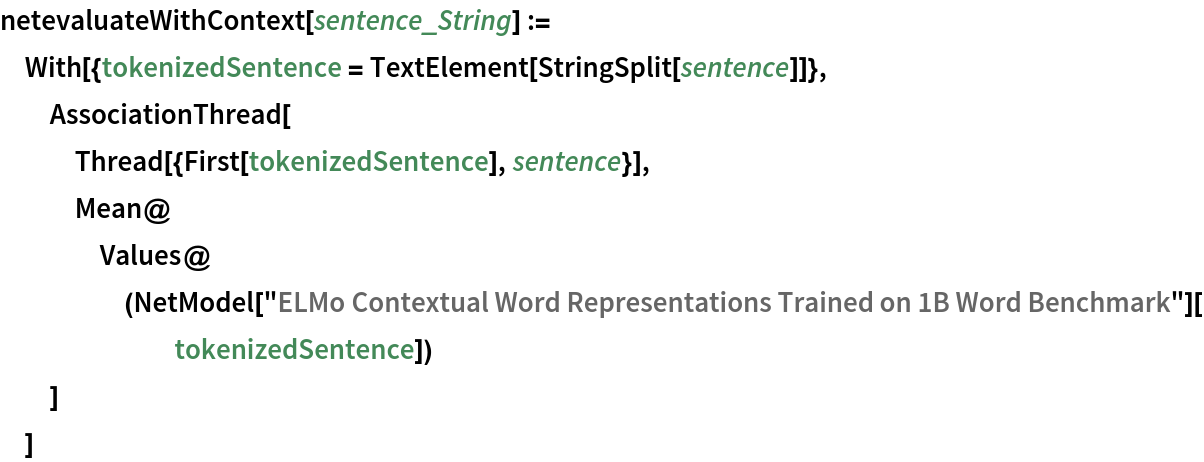Check the result on a sentence:

 In:=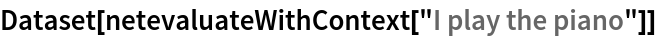Out=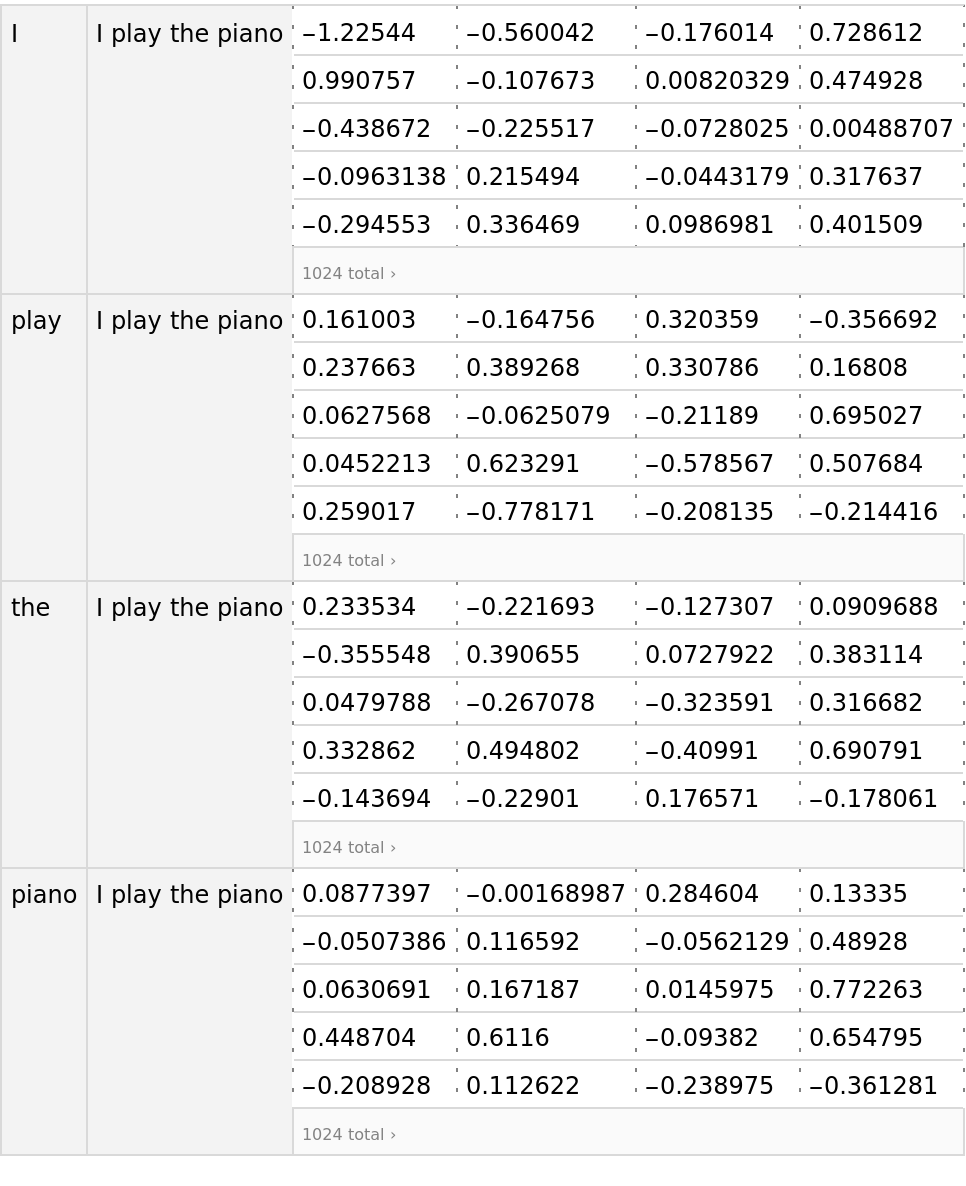Define a function to find the nearest word in context in a set of sentences, for a given word in context:

 In:=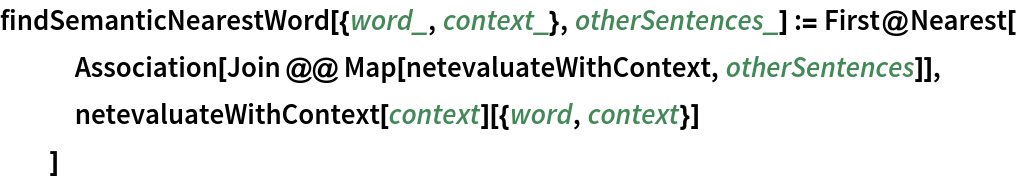Find the semantically nearest word to the word "play" in "I play the piano":

 In:=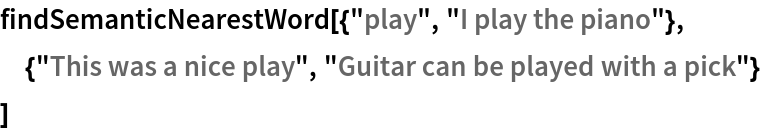Out=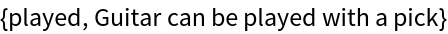Find the semantically nearest word to the word "set" in "The set of values higher than a threshold":

 In:=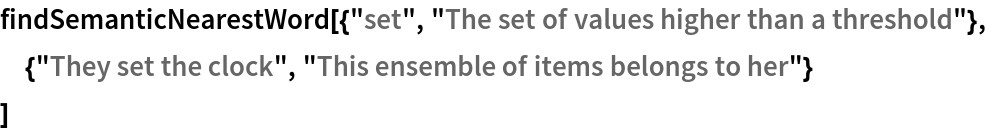Out=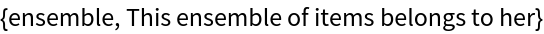### Train a model with the word embeddings

Take text-processing dataset:

 In:=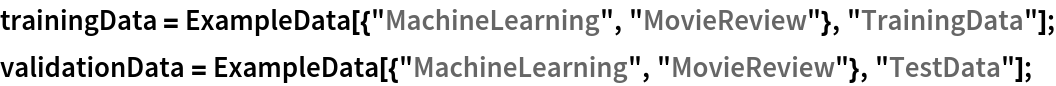Pre-compute the ELMo vectors on the training and the validation dataset (if available, GPU is recommended):

 In:=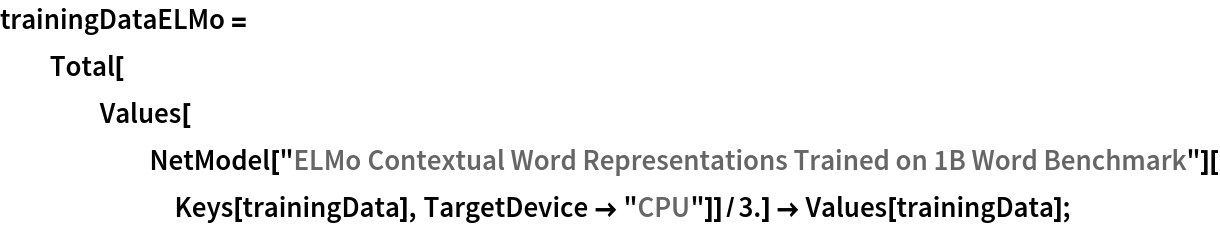In:=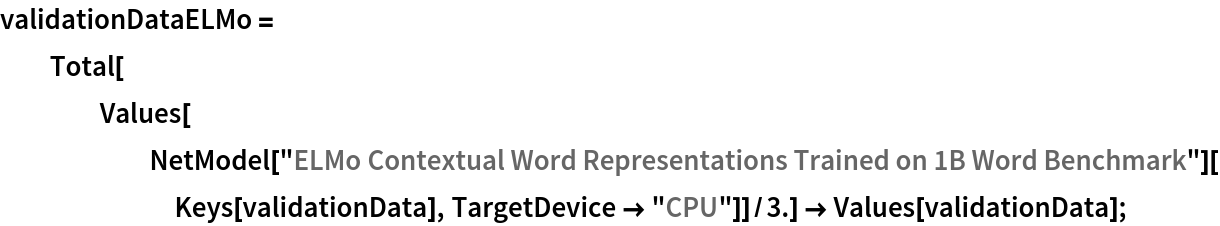Define a network that takes word vectors instead of strings for the text-processing task:

 In:=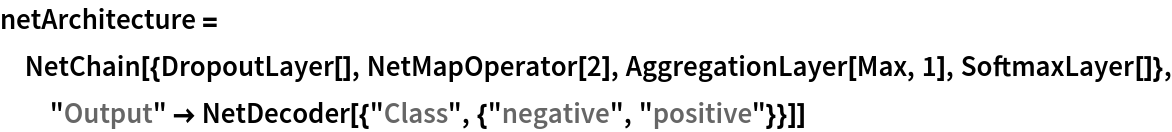Out=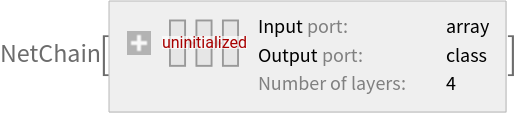Train the network on the pre-computed ELMo vectors:

 In:=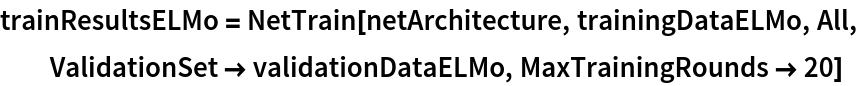Out=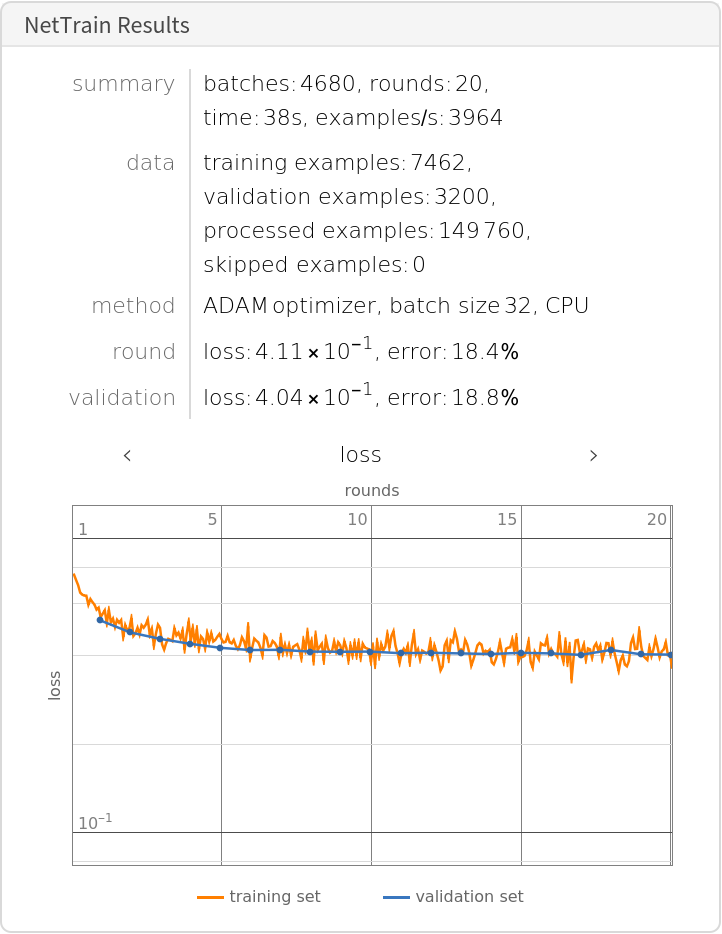Check the classification error rate on the validation data:

 In:=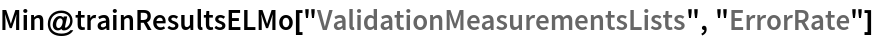Out=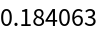Compare the results with the performance of the same model trained on context-independent embeddings:

 In:=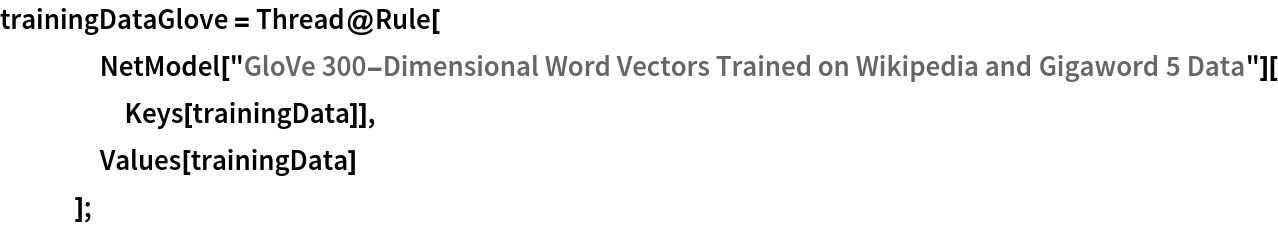In:=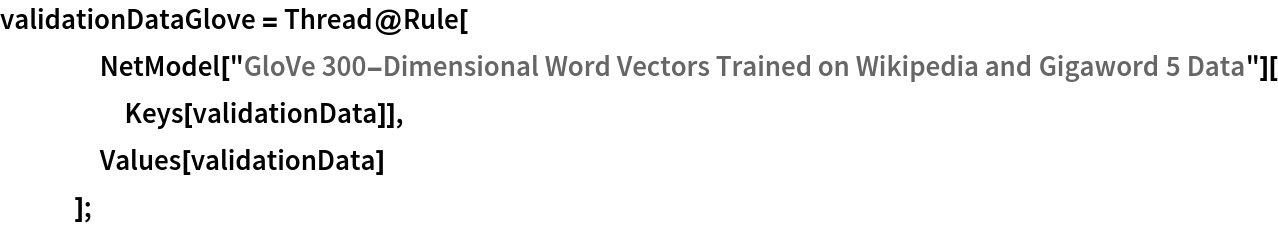In:=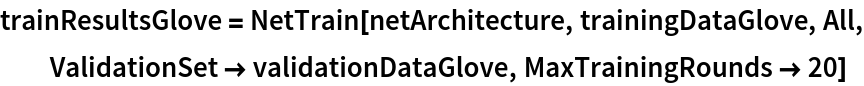Out=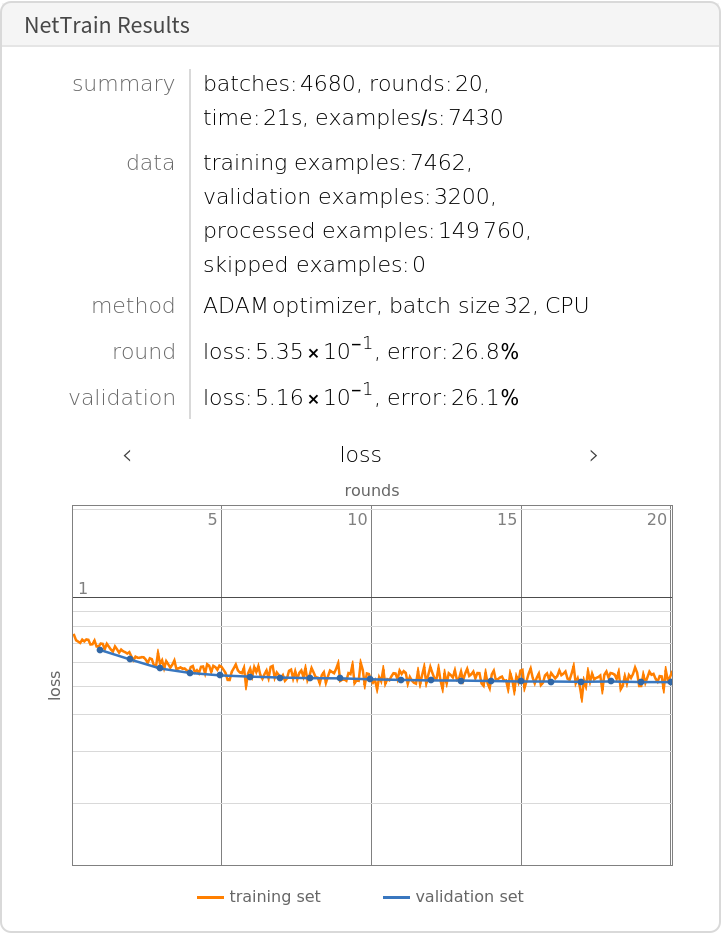In:=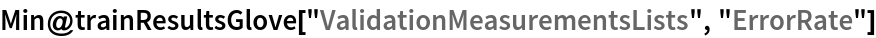Out=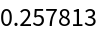### Net information

Inspect the number of parameters of all arrays in the net:

 In:=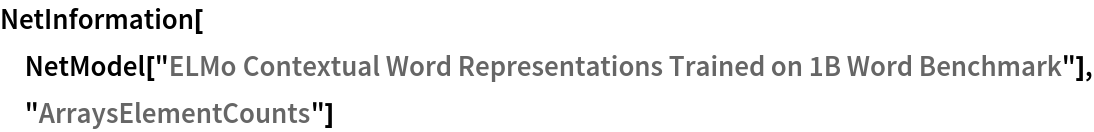Out=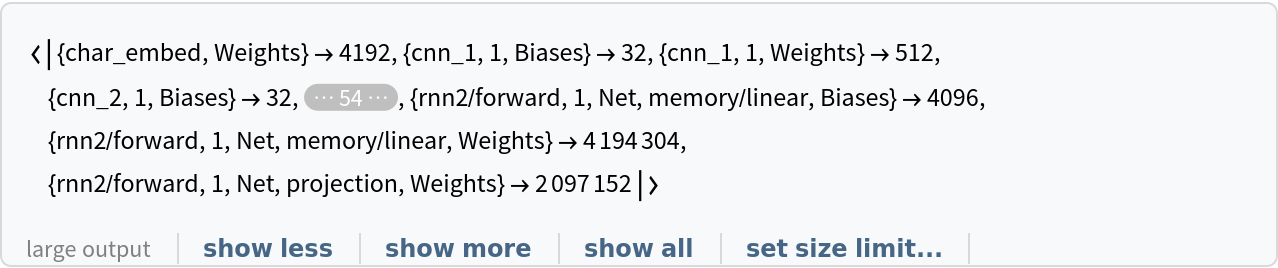Obtain the total number of parameters:

 In:=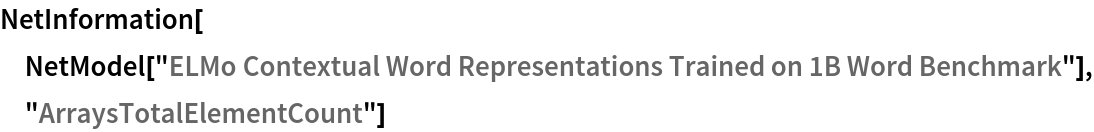Out=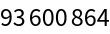Obtain the layer type counts:

 In:=Out=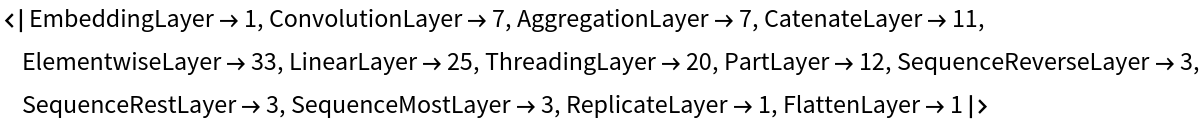Display the summary graphic:

 In:=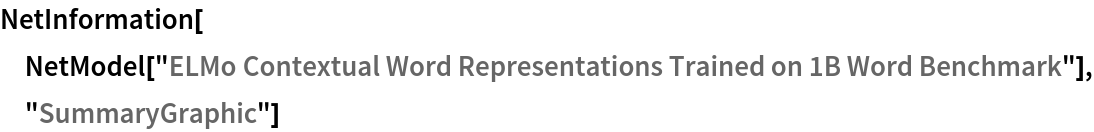Out=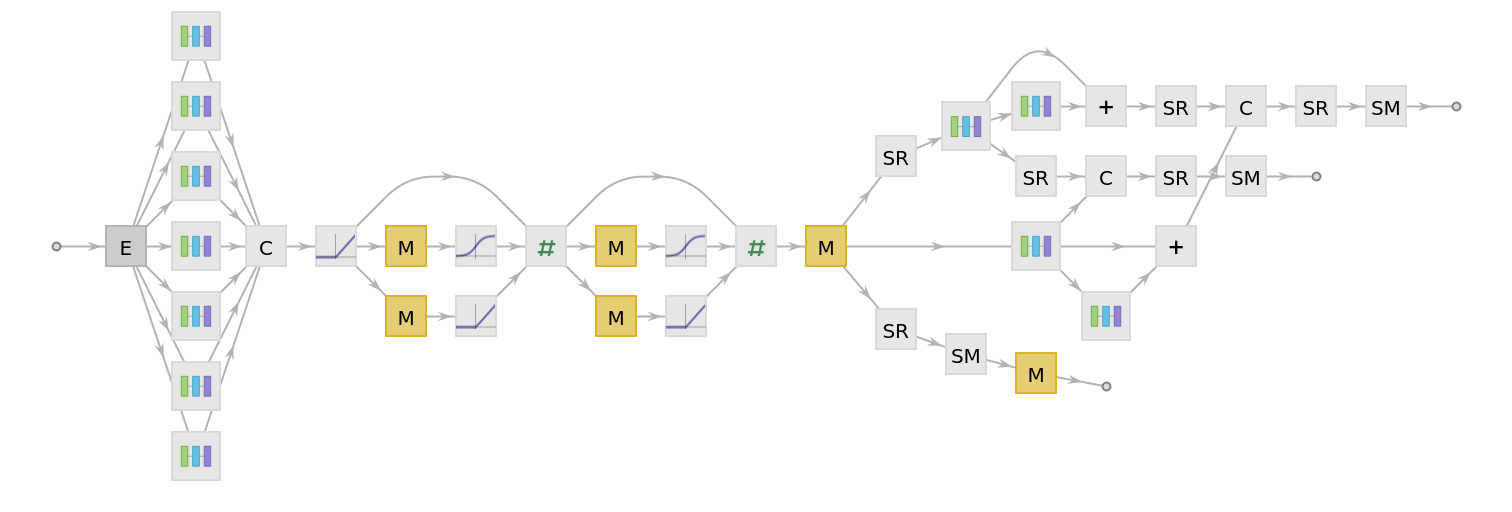### Export to MXNet

Export the net into a format that can be opened in MXNet:

 In:=Out=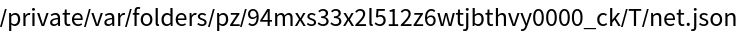Export also creates a net.params file containing parameters:

 In:=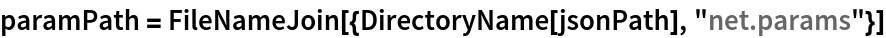Out=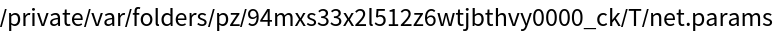Get the size of the parameter file:

 In:=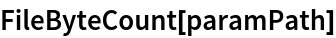Out=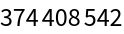The size is similar to the byte count of the resource object:

 In:=Out=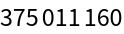## Requirements

Wolfram Language 11.3 (March 2018) or above

## Reference

• M. E. Peters, M. Neumann, M. Iyyer, M. Gardner, C. Clark, K. Lee, L. Zettlemoyer, "Deep Contextualized Word Representations," arXiv:1802.05365 NAACL(2018)
• Available from: http://allennlp.org/elmo